FREE ASSISTANCE FOR THE INQUISITIVE PEOPLE
Tutorial Topics
Newtons law of motion - 1st Law
Newtons law of motion - 2nd Law - Rest And Motion
1600    Arnab De    20/08/2018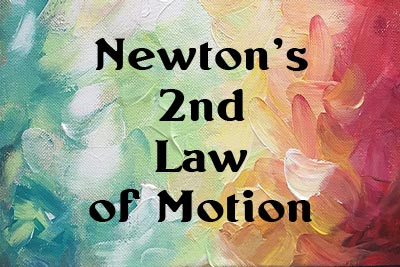Sir Isaac Newton was an English scientist. In the year 1686 he put forwarded three laws about the motion, which are known as Newton’s laws of motion. Here we discuss about the second law of Newton’s laws of motion.

The second newton’s law of motion

The liner momentum of a body of mass m moving with velocity v is define as the product of mass and velocity.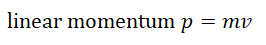Def : The rate of change of momentum of a body is directly proportional to the net external force applied on it and the change in momentum takes place in the direction in which the force is applied.

We know from the 2nd law of motion

1. Concept of momentum
2. Unit of force
3. Measurement force

### Concept of momentum

Def. The product of mass and velocity of a body produce a motion of the body which is known as momentum of the body.

Therefore, momentum is the product of the mass and velocity. It is a vector quantity because it has both value and direction. The direction of momentum is the same as the direction of velocity.

#### Unit of the momentum

S.I method : kg mtr/s

C.G.S method : gm cm/s

F.P.S method : pound foot/s

If two bus, one with full of passenger, another one is empty. Both are run in the same velocity then first one must be faster than the second one because the momentum of the first bus is bigger than the second one, as mass of the first one is greater than the second. Therefore, much more force need to stop the first bus.

### Unit of force

The SI unit of the force is newton. The symbol of newton is N.

In CGS system, the unit of force is dyne.

Relationship between newton and dyne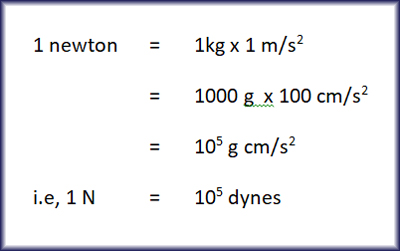### Gravitational Unit of force

The SI unit of the force is newton. The symbol of newton is N.

In CGS system, the unit of force is dyne.

### Measurement force

We know, mass of a body new change. i.e. mas cannot increase or decrease, it is a constant property of any body. That is, change of momentum of a body is depending on the change of velocity of the body. Increasing of velocity means apply the force towards the direction of moving body (i.e. Acceleration). If we apply the force towards the opposite direction of the motion, the velocity decrease (i.e. Retardation).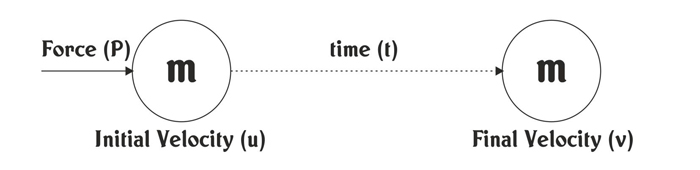Now, let initial velocity of a body is u and final velocity of the body is v. now we apply a force P for the t time and the mass of the body is m.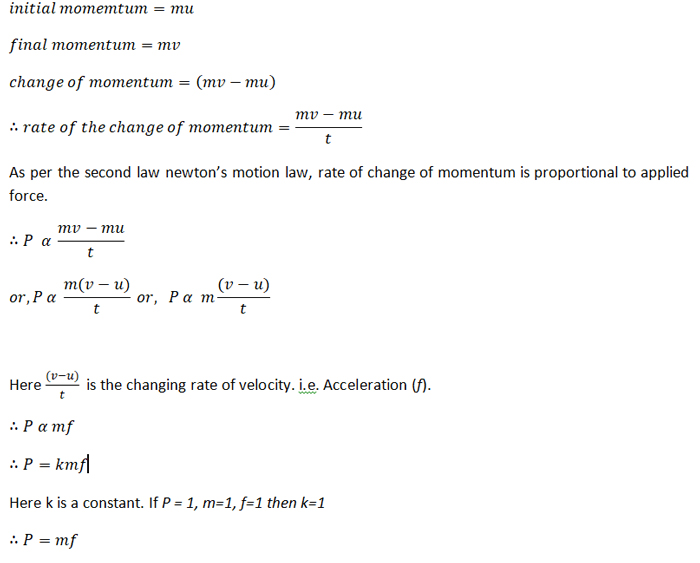Therefore, force= mass x acceleration. This is the equation to find out the force.

Newtons law of motion - 1st Law
Author DetailsArnab De
I have over 16 years of experience working as an IT professional, ranging from teaching at my own institute to being a computer faculty at different leading institute across Kolkata. I also work as a web developer and designer, having worked for renowned companies and brand. Through tutorialathome, I wish to share my years of knowledge with the readers.
Enter New Comment
Comment History
No Comment Found Yet.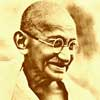By education, I mean all-around drawing of the best in child and man in body, mind and sprit.
Mahatma Gandhi
1950
25.81
Today So Far
Total View (Lakh)